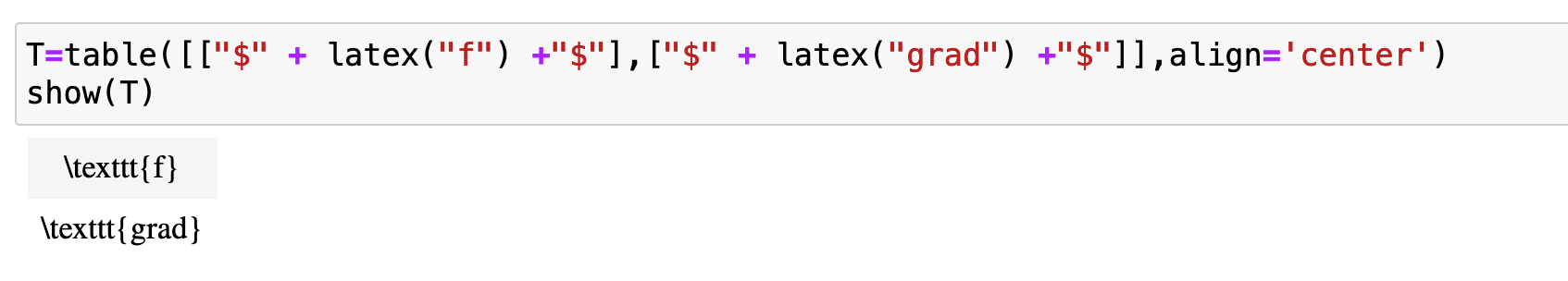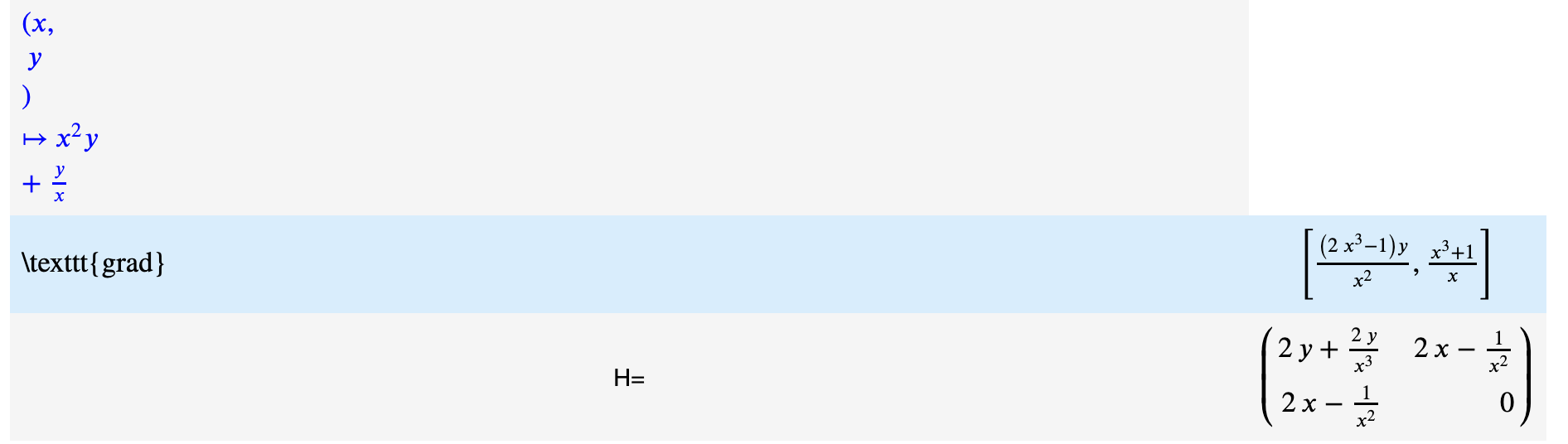# nice font in "table"hi

i have this code :

show("grad")
show(T)


the result of the first line is "grad" in some beautiful font

but the result of the show(T) is "grad" in some other font

can i display the show(T) with the font that Sagemaths uses when running show("grad") ?

Vinz

edit retag close merge delete

Sort by » oldest newest most votedLike this:

T=table([["$" + latex("grad") +"$"]])

morethank you ; i can't manage to have the good result see imagei also have a problem of alignment in table :with this code :

display_f=html(r"$\color{blue}{" + latex(f) + r"}$")
T=table([[display_f],["$" + latex("grad") +"$",grad(F)[:]],["H=",H]],frame=True,align='center')
show(T)

more